# Class 12 Maths NCERT Solutions for Chapter 6 Application of Derivatives Miscellaneous Exercise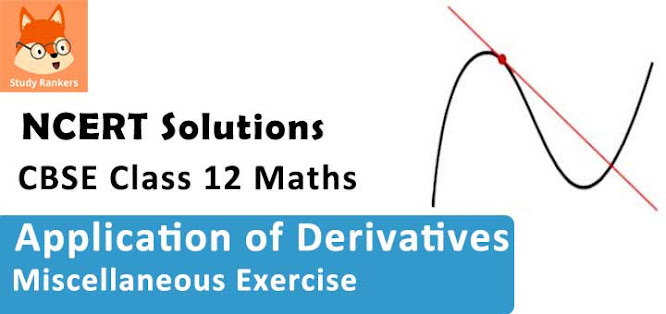### Application of Derivatives Miscellaneous Exercise Solutions

1. Using differentials, find the approximate value of each of the following.
(i) (17/81)1/4
(ii) (33)1/5

Solution

(i) Consider y = x1/4 . Let x = 16/81 and Δx = 1/81 .
Then, Δy = (x + Δx)1/4 - x1/4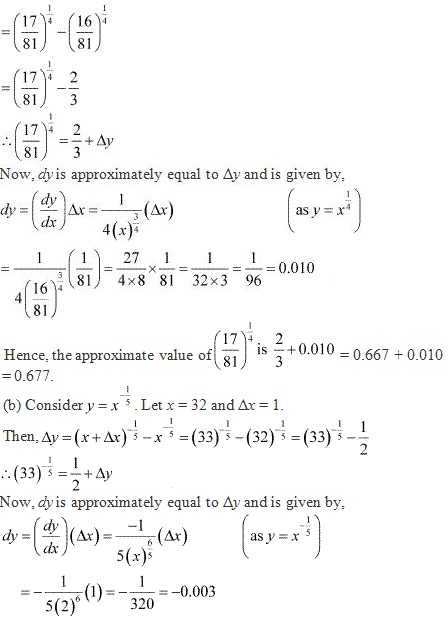Hence, the approximate value of (33)-1/5 is 1/2 + (-0.003)
= 0.5 - 0.003 = 0.497

2. Show that the function given by f(x) = logx/x has maximum at x = e.

Solution

The given function is f(x) = log x/ x.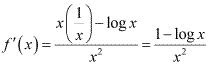Now, f '(x) = 0
⇒ 1 - log x = 0
⇒ log x = 1
⇒ log x = log e
⇒ x = e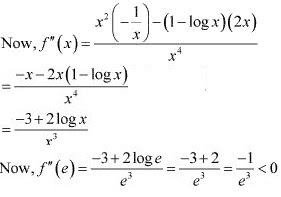Therefore, by second derivative test, f is the maximum at x = e.

3. The two equal sides of an isosceles triangle with fixed base b are decreasing at the rate of 3 cm per second. How fast is the area decreasing when the two equal sides are equal to the base?

Solution

Let ∆ABC be isosceles where BC is the base of fixed length b.
Let the length of the two equal sides of ∆ABC be a.Now, in ∆ADC, by applying the Pythagoras theorem, we have :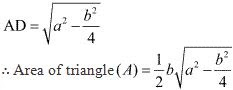The rate of change of the area with respect to time (t) is given by,It is given that the two equal sides of the triangle are decreasing at the rate of  3 cm per second.
∴ da/dt = -3 cm/s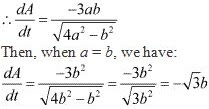Hence, it the two equal sides are equal to the base, then the area of the triangle is decreasing at the rate of √3b cm2/s.

4. Find the equation of the normal to curve y2 = 4x at the point (1, 2).

Solution

The equation of the given curve is  y2 = 4x .
Differentiating with respect to x, we have :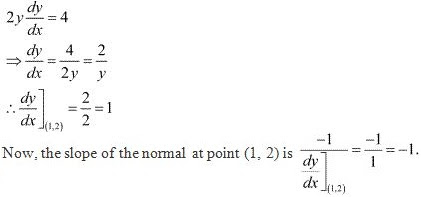∴ Equation of the normal at (1, 2) is y - 2 = -1(x - 1).
⇒ y - 2 = -x + 1
⇒ x+ y - 3 = 0
OR
If we consider the equation of the curve as x2 = 4y
Differentiating with respect to x, we have :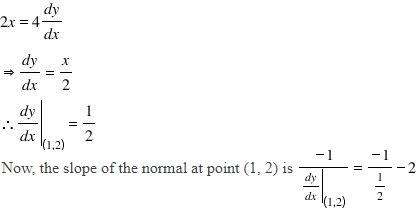∴ Equation of the normal at (1, 2) is y - 2 = -2(x - 1)
⇒ y - 2 = -2x + 2
⇒ 2x + y - 4 = 0

5. Show that the normal at any point θ to the curve x = a cosθ + a θ sinθ, y = a sinθ – aθ cosθ is at a constant distance from the origin.

Solution

We have x = a cos θ + a θ sin θ.
∴ dx/dθ = -a sin θ + a sin θ + aθ cos θ = aθ cosθ
y = a sinθ - aθ cosθ
∴ dy/dθ = a cos θ - a cos θ + aθ sinθ = aθ sin θ
∴ dy/dx = dy/dθ . dθ/dx = aθ sinθ/aθ cosθ = tan θ
∴ Slope of the normal at any point θ is -1/tan θ.
The equation of the normal at a given point(x, y) is given by,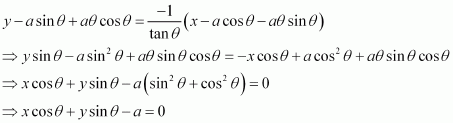Now, the perpendicular distance of the normal from the origin is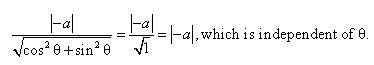Hence, the perpendicular distance of the normal from the origin is constant.

6. Find the intervals in which the function f given by f(x) = (4 sin x - 2x - x cos x)/(2 + cos x) is
(i) Increasing
(ii) decreasing

Solution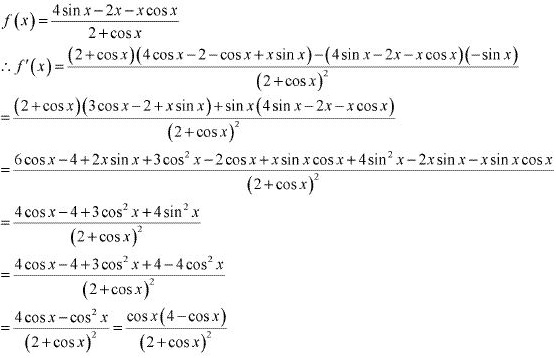N f'(x) = 0
⇒ cos x = 0 or cos x = 4
But, cos x ≠ 4
∴ cos x = 0
⇒ x = π/2, 3π/2
Now, x = π/2 and x = 3π/2 divides (0, 2π) into three disjoint intervals i.e.,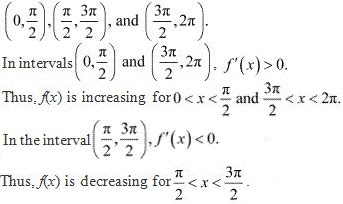7. Find the intervals in which the function f given by f(x) = x3 +1/x3  × ! = 0, is
(i) increasing
(ii) decreasing
Solution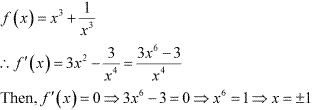Now, the points x = 1 and x = -1 divide the real line into three disjoint intervals i.e., (-∞, -1), (-1, 1) and (1, ∞).
In intervals (-∞, -1) and (1, ∞) i.e., when x < -1 and x > 1, f '(x) > 0.
Thus, when x < -1 and x > 1,  f is increasing.
In interval (-1, 1) i.e, when -1 < x < 1, f '(x) < 0 .
Thus, when -1 < x < 1 f is decreasing.

8. Find the maximum area of an isosceles triangle inscribed in the ellipse x2/a2 + y2/b2 = 1 with its vertex at one end of the major axis.
Solution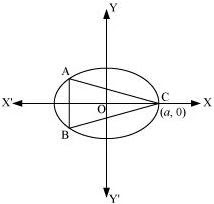The given ellipse is  x2/a2 + y2/b2 = 1 .
Let the major axis be along the x - axis.
Let ABC be the triangle inscribed in the ellipse where vertex C is at (a, 0).
Since the ellipse is symmetrical with respect to the x - axis and y - axis, we can assume the coordinates of A to be (−x1 , y1 ) and the coordinates of B to be (−x1 , y1 ) .
Now, we have y1 =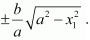∴ Coordinates of A are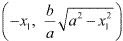and the coordinates of B are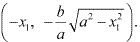As the point (x1 , y1 ) lies on the ellipse, the area of triangle ABC (A) is given by,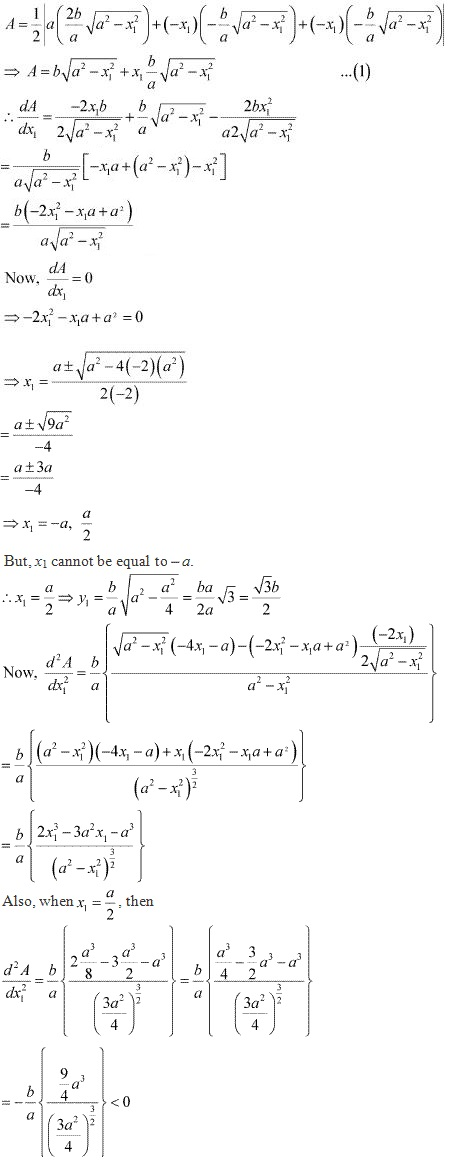Thus, the area is the maximum when x1 = a/2.
∴ Maximum area of the triangle is given by,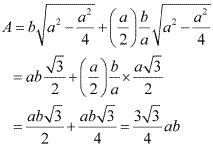9. A tank with rectangular base and rectangular sides, open at the top is to the constructed so that its depth is 2 m and volume is 8 m3. If building of tank cost 70 per square metre for the base and Rs 45 per square matre for sides, what is the cost of least expensive tank?
Solution

Let lb, and h represent the length, breadth, and height of the tank respectively.
Then, we have height (h) = 2 m
Volume of the tank = 8m3
Volume of the tank = l × b × h
∴ 8 = × b × 2
⇒ lb = 4
⇒ b = 4/l
Now, area of the base = lb = 4
Area of the 4 walls (A) = 2h(+ b)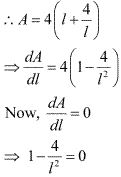l2 = 4
l = ± 2
However, the length cannot be negative.
Therefore, we have l = 2.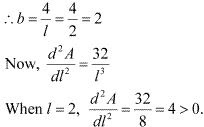Thus, by second derivative test, the area is the minimum when l = 2.
We have b = h = 2.
∴Cost of building the base = Rs 70 × (lb) = Rs 70 (4) = Rs 280
Cost of building the walls = Rs 2h (l + b) × 45 = Rs 90 (2) (2 + 2)
= Rs 8 (90) = Rs 720
Required total cost = Rs (280 + 720) = Rs 1000
Hence, the total cost of the tank will be Rs 1000.

10. The sum of the perimeter of a circle and square is k, where k is some constant. Prove that the sum of their areas is least when the side of square is double the radius of the circle.

Solution

Let r be the radius of the circle and a be the side of the square.
Then, we have :
2πr + 4a = k (where k is constant)
⇒ a = (k - 2πr)/4
The sum of the areas of the circle and the square (A) is given by,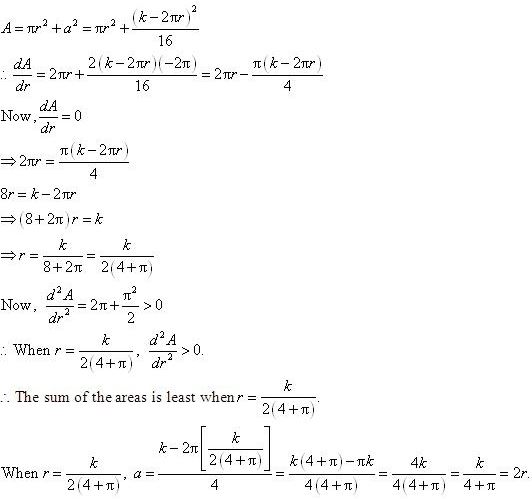Hence, it has been proved that the sum of their areas is least when the side of the square is double the radius of the circle.

11. A window is in the form of rectangle surmounted by a semicircular opening. The total perimeter of the window is 10 m. Find the dimensions of the window to admit maximum light through the whole opening.

Solution

Let x and y be the length and breadth of the rectangular window.
Radius of the semicircular opening = x/2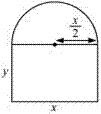It is given that the perimeter of the window is 10 m.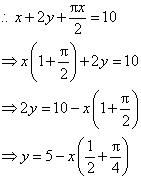∴ Area of the window (A) is given by,Therefore, by second derivative test, the area is the maximum when length x = 20/(π + 4) m.
Now,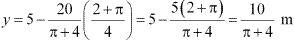Hence, the required dimensions of the window to admit maximum light is given by length = 20/(π + 4) m and breadth = 10/(π + 4) m.

12. A point on the hypotenuse of a triangle is at distance and b from the sides of the triangle.
Show that the minimum length of the hypotenuse is (a2/3 + b2/3 )3/2

Solution

Let ΔABC be right - angled at B. Let AB = x and BC = y.
Let P be a point on the hypotenuse of the triangle such that P is at a distance of a and b from the sides AB and BC respectively.
Let ∠C = θ .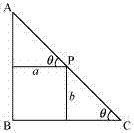We have,
AC = √(x2 + y2 )
Now,
PC = b cosec θ
And, AP = a sec θ
∴ AC = AP + PC
⇒ AC = b cosec θ + a sec θ ...(1)
∴ d(AC)/dθ = -bcosecθ cotθ + asecθ tanθ
∴ d(AC)/dθ = 0
⇒ a secθ tanθ = bcosecθ cotθ
⇒ a/cos θ . sinθ/cosθ = (b/sinθ)(cosθ/sinθ)
⇒ a sin3 θ = b cos3 θ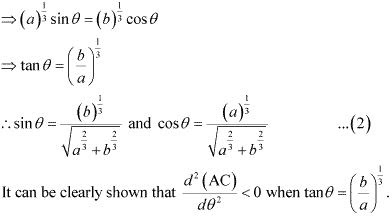Therefore, by second derivative test, the length of the hypotenuse is the maximum when
tan θ = (b/a)1/3 .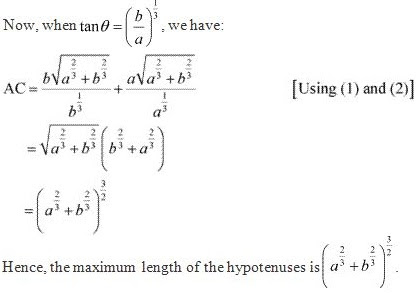13. Find the points at which the function f given by f (x) = (x – 2)4 (x + 1)3 has
(i) local maxima
(ii) local minima
(iii) point of inflexion

Solution

The given function is f(x) = (x – 2)4 (x + 1)3 .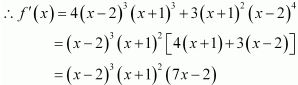Now, f '(x) = 0
⇒ x = -1 and x = 2/7 or x = 2
Now, for values of close to 2/7and to the left of 2/7, f '(x) > 0 . Also, for values of x close to 2/7 and to the right of 2/7, f'(x) < 0.
Thus, x = 2/7 is the point of local maxima.
Now, for values of x close to 2 and to the left of 2, f'(x) <0. Also, for values of x close to 2 and to the right of 2, f'(x) > 0.
Thus, x = 2 is the point of local minima.
Now, as the value of x varies through −1, f'(x) does not changes its sign.
Thus, x = −1 is the point of inflexion.

14. Find the absolute maximum and minimum values of the function f given by f (x) = cos2 x + sin x, x ∈ [0, π].

Solution

f(x) = cos2 x + sin x
f'(x) = 2cosx(- sinx) + cos x
= -2sin x cos x + cos x
Now, f '(x) = 0
⇒ 2 sinx cos x = cos x ⇒ cos x(2sin x - 1) = 0
⇒ sin x = 1/2 or cos x = 0
⇒ x = π/6, or π/6 as x ∊ [0, π]
Now, evaluating the value of f at critical points x = π/2 and x = π/6 and at the end points of the interval [0, π] (i,e., at x = 0 and x = π) , we have :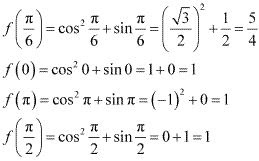Hence, the absolute maximum value of f is 5/4 occurring at x = π/6 and the absolute minimum value of f is 1 occurring at x = 0, π/2 and π.

15. Show that the altitude of the right circular cone of maximum volume that can be inscribed in a sphere of radius r 4r/3.

Solution

Let R and h be the radius and the height of the cone respectively.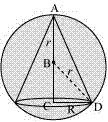The volume (V) of the cone is given by,
V = (1/3)πR2 h
Now, from the right triangle BCD, we have :
BC = √(r2 - R2 )
∴ h = r + √(r2 - R2 )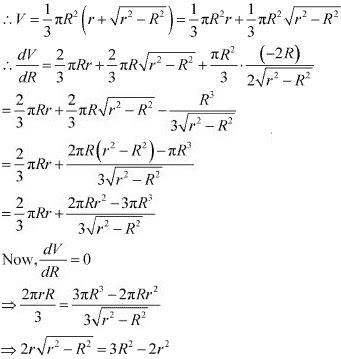⇒ 4r2 (r2 - R2 ) =  (3R2 - 2r2 )2
⇒ 4r4 - 4r2 R2 = 9R4 + 4r4 - 12R2 r2
⇒ 9R4 - 8r2 R2 = 0
⇒ 9R2 = 8r2
⇒ R2 = 8r2/9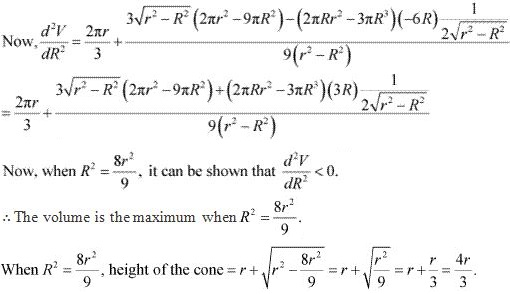Hence, it can be seen that the altitude of the right circular cone of maximum volume that can be inscribed in a sphere of radius r is 4r/3.

16. Let f be a function defined on [ab] such that f '(x) > 0, for all x ∈ (ab). Then prove that f is an increasing function on (ab).

Solution

Since f '(x) > 0 on (a, b)
∴ f is a differentiable function (a, b)
Also every differentible function is continuous, therefore f is continuous on (a, b)
Let x1 , x2 ∊ (a, b) and x2 > x1 then by LMV theorem, there exist c ∊ (a, b) s.t.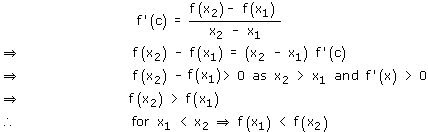⇒ f is an increasing function.

17. Show that the height of the cylinder of maximum volume, which can be inscribed in a sphere of radius R is 2R√3. Also find the maximum volume.

Solution

A sphere of fixed radius (R) is given.
Let r and h be the radius and the height of the cylinder respectively.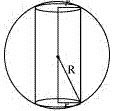From the given figure, we have h = 2√(R2 - r2 )
The volume (V)  of the cylinder is given by,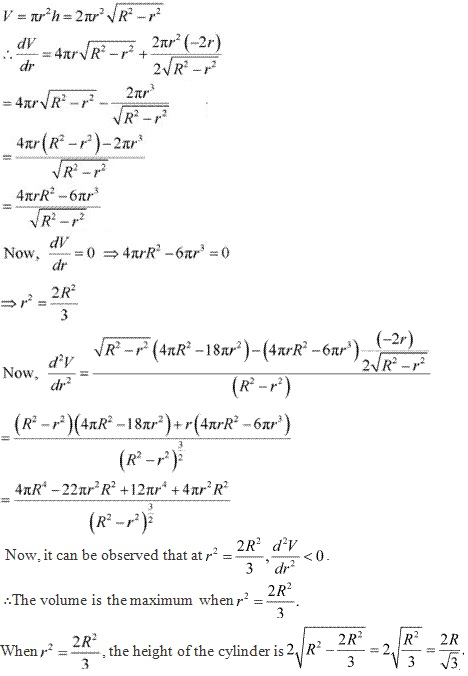Hence, the volume of the cylinder is the maximum when the height of the cylinder is 2R/√3.

18. Show that height of the cylinder of greatest volume which can be inscribed in a right circular cone of height h and semi vertical angle α is one-third that of the cone and the greatest volume of cylinder is (4/27)πh3 tan2α.

Solution

The given right circular cone of fixed height (h) and semi - vertical angle (a) can be drawn as :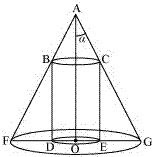Here, a cylinder of radius R and height H is inscribed in the cone.
Then, ∠GAO = a, OG = r, OA = h, OE = R, and CE = H.
We have,
r = h tan a
Now, since ΔAOG is similar to ΔCEG, we have :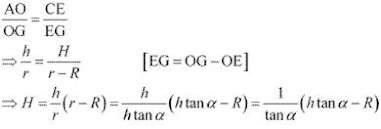Now, the volume (V) of the cylinder is given by,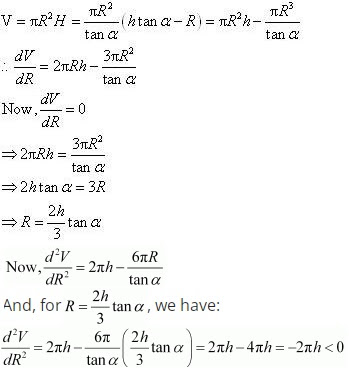∴ By second derivative test, the volume of the cylinder is the greatest when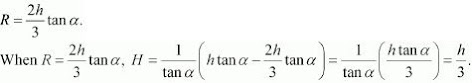Thus, the height of the cylinder is one-third the height of the cone when the volume of the cylinder is the greatest.
Now, the maximum volume of the cylinder can be obtained as: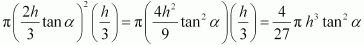Hence, the given result is proved.

19. A cylindrical tank of radius 10 m is being filled with wheat at the rate of 314 cubic mere per hour. Then the depth of the wheat is increasing at the rate of
(A) 1 m/h
(B) 0.1 m/h
(C) 1.1 m/h
(D) 0.5 m/h

Solution

Then, volume (V) of the cylinder is given by,
V = π (radius)2 × height
= π(10)2 h  (radius = 10 m)
= 100πh
Differentiating with respect to time t, we have :
DV/dt = 100π(dh/dt)
The tank is being filled with wheat at the rate of 314 cubic metres per hour.
∴ dV/dt = 314 m3 /h
Thus, we have :
314 = 100π(dh/dt)
⇒ dh/dt = 314/[100(3.14)] = 314/314 = 1
Hence, the depth of wheat is increasing at the rate of 1 m/h.

20. The slope of the tangent to the curve x = t2 + 3t – 8, y = 2t2 – 2t – 5 at the point (2,– 1) is
(A) 22/7
(B) 6/7
(C) 7/6
(D) -6/7

Solution

The given curve is x = t2 + 3t – 8 and y = 2t2 – 2t – 5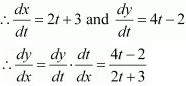The given point is (2, −1).
At x = 2, we have: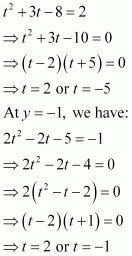The common value of t is 2.
Hence, the slope of the tangent to the given curve at point (2, −1) is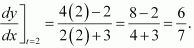21. The line y = mx + 1 is a tangent to the curve y2 = 4x if the value of m is
(A) 1
(B) 2
(C) 3
(D) 1/2

Solution

The equation of the tangent to the given curve is y = mx + 1 .
Now, substituting y = mx + 1 in y2 = 4x, we get :
⇒ (mx + 1)2 = 4x
⇒ m2 x2 + 1 + 2mx - 4x = 0
⇒ m2 x2 + x(2m - 4) + 1 = 0  ...(i)
Since a tangent touches the curve at one point, the roots of equation (i) must be equal.
Therefore, we have :
Discriminant = 0
(2m - 4)2 - 4(m2)(1) = 0
⇒ 4m2 + 16 - 16m - 4m2 = 0
⇒ 16 - 16m = 0
⇒ m = 1
Hence, the required value of m is 1.

22. The normal at the point (1, 1) on the curve 2y + x2 = 3 is
(A) x + y = 0
(B) x − = 0
(C) x + y + 1 = 0
(D) − y = 1

Solution

The equation of the given curve is 2y + x2 = 3.
Differentiating with respect to x, we have :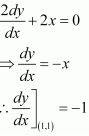The slope of the normal to the given curve at point (1, 1) is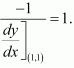Hence, the equation of the normal to the given curve at (1, 1) is given as :
⇒ y - 1 = 1(x - 1)
⇒ y - 1 = x - 1
⇒ x - y = 0

23. The normal to the curve x2 = 4y passing (1, 2) is
(A) x + y = 3
(B) x − y = 3
(C) x + = 1
(D) x − = 1

Solution

The equation of the given curve is x2 = 4y.
Differentiating with respect to x, we have :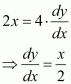The slope of the normal to the given curve at point (h, k) is given  by,∴ Equation of the normal at point (h, k) is given as :
y - k = (-2/h) (x - h)
Now, it is given that the normal passes through the point (1, 2).
Therefore, we have :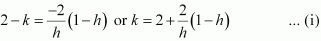Since (h, k) lies on the curve x2 = 4y ,  we have h2 = 4k
⇒ k = h2 /4
From equation (i), we have :Hence, the equation of the normal is given as :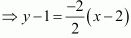⇒ y - 1 = -(x - 2)
⇒ x + y = 3

24. The points on the curve 9y2 = x3, where the normal to the curve makes equal intercepts with the axes are

(A) (4,  ± 8/3)
(B) (4, -8/3)
(C) (4, ± 3/8)
(D) (±4, 3/8)

Solution

The equation of the given curve is 9y2 = x3.
Differentiating with respect to x, we have: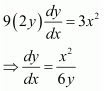The slope of the normal to the given curve at point (x1 , y1 ) is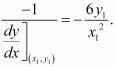∴ The equation of the normal to the curve at (x1 , y1 ) is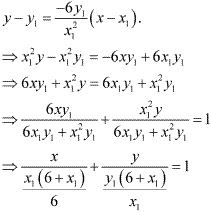It is given that the normal makes equal intercepts with the axes.
Therefore, we have: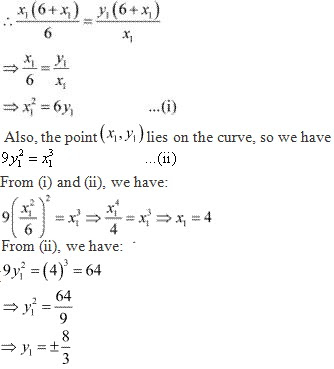Hence, the required points are (4, ± 8/3).